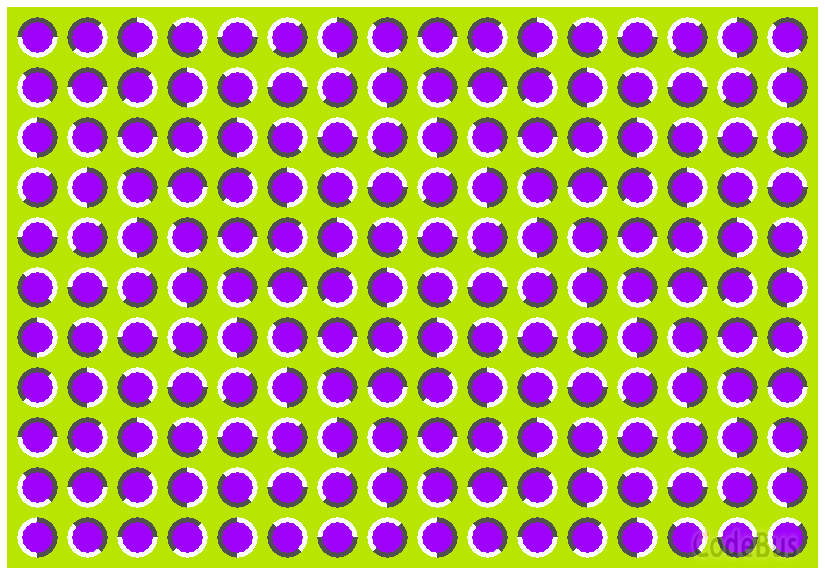游戏介绍

• 鼠标左键拖动图形；
• 鼠标右键使七巧板顺时针旋转 45 度；
• 按住左键时按空格可以让四边形轴对称翻转。

完整游戏源代码

编译环境

VisualStudio 2019，EasyX_20200902

文件描述

Point.h

...三、完整代码如下

///////////////////////////////////////////////////
// 程序名称：视觉错觉艺术图
// 编译环境：VS2019， EasyX_20200902
// 作　　者：梦萤花火
// 最后修改：2021-1-24
//
#include <graphics.h>
#include <conio.h>

int x;								// X轴坐标
int y;								// y轴坐标
int g_multiples = 0;				// 倍数：作用是对弧度角进行改变
float g_PI = 3.1415927;				// 圆周率

// 实施画单个完整小球
...c.re = 0.45, c.im = -0.1428;
c.re = 0.285, c.im = 0.01;
c.re = 0.285, c.im = 0;
c.re = -0.8, c.im = 0.156;
c.re = -0.835, c.im = -0.2321;
c.re = -0.70176, c.im = -0.3842;

...HSLtoRGB((float)((k<<5) % 360), 1.0, 0.5)

// 程序名称：分形学 - Mandelbrot Set (曼德布洛特集)
// 编译环境：Visual C++ 6.0，EasyX_20200902
// 最后更新：2010-9-9
//
#include <graphics.h>
#include <conio.h>

///////////////////////////////////////////
...

////////////////////////////////////////////
// 程序名称：打字母游戏
// 编译环境：Visual C++ 6.0 / 2010，EasyX_20200902
// 程序编写：yangw80 <yw80@qq.com>
// 发布日期：2010-8-26
//
#include <graphics.h>
#include <conio.h>
#include <time.h>

// 欢迎界面
void welcome()
{
// 输出屏幕提示
cleardevice();
settextcolor(YELLOW);
sett
...// 程序名称：彩虹
// 编译环境：Visual C++ 6.0，EasyX_20200902
// 最后更新：2010-8-25
//
#include <graphics.h>
#include <conio.h>

int main()
{
float H, S, L;

initgraph(640, 480);

// 画渐变的天空(通过亮度逐渐增加)
H = 190;	// 色相
S = 1;		// 饱和度
L = 0.7f;	// 亮度
for(int y = 0; y < 480; y++)
{
L += 0.0005f;
setlinecolor( HSLtoRGB(H, S, L) );
line(0, y, 639, y);
}

// 画彩虹(通过色相逐渐增加)
H =
...

// 程序名称：星空
// 编译环境：Visual C++ 6.0，EasyX_20200902
// 最后更新：2009-2-22
//
#include <graphics.h>
#include <time.h>
#include <conio.h>

#define MAXSTAR 200	// 星星总数

struct STAR
{
double x;
int y;
double step;
int color;
};

STAR star[MAXSTAR];

// 初始化星星
void InitStar(int i)
{
star[i].x = 0;
star[i].y = rand() % 480;
star[i].step = (rand() % 5000) / 1000.0 + 1;
star[i].color = (int)(star[i].step * 255 / 6.0 + 0.5);	//
...

// 程序名称：字符阵
// 编译环境：Visual C++ 6.0 / 2010，EasyX_20200902
// 发布日期：2009-2-22
//
#include <graphics.h>
#include <time.h>
#include <conio.h>

int main()
{
// 设置随机函数种子
srand((unsigned)time(NULL));

// 初始化图形模式
initgraph(640, 480);

int x, y;
TCHAR c;

settextstyle(16, 8, _T("Courier"));	// 设置文字样式
settextcolor(GREEN);				// 设置文字颜色
setlinecolor(BLACK);				// 设置画线颜色

while (!_kbhit())
{
for (int i = 0; i < 479;
...